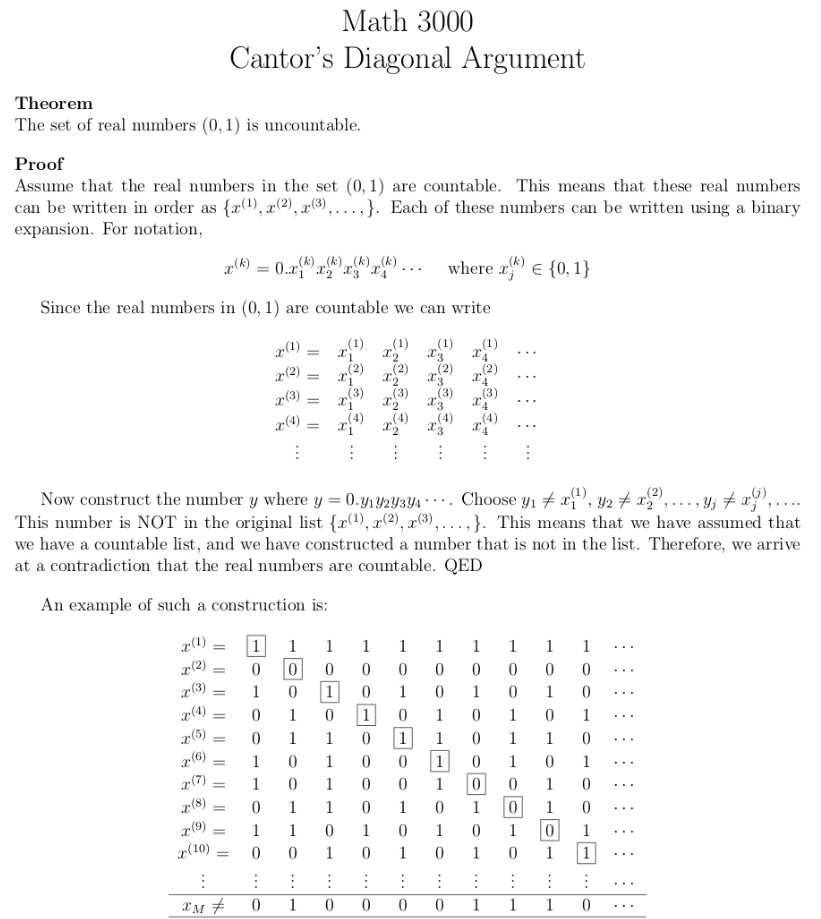# [Mirror] Cantor was Wrong: debunking the infinite set hierarchy

2019 Apr 01 See all posts

[Mirror] Cantor was Wrong: debunking the infinite set hierarchy

This is a mirror of the post at https://medium.com/@VitalikButerin/cantor-was-wrong-debunking-the-infinite-set-hierarchy-e9ba5015102.

By Vitalik Buterin, PhD at University of Basel

A common strand of mathematics argues that, rather than being one single kind of infinity, there are actually an infinite hierarchy of different levels of infinity. Whereas the size of the set of integers is just plain infinite, and the set of rational numbers is just as big as the integers (because you can map every rational number to an integer by interleaving the digits of its numerator and denominator, eg. $$0.456456456.... = \frac{456}{999} = \frac{152}{333} \rightarrow 135323$$), the size of the set of real numbers is some kind of even bigger infinity, because there is no way to make a similar mapping from real numbers to the integers.

First of all, I should note that it's relatively easy to see that the claim that there is no mapping is false. Here's a simple mapping. For a given real number, give me a (deterministic) python program that will print out digits of it (eg. for π, that might be a program that calculates better and better approximations using the infinite series $$\pi = 4 - \frac{4}{3} + \frac{4}{5} - \frac{4}{7} + ...$$). I can convert the program into a number (using n = int.from_bytes(open('program.py').read(), 'big')) and then output the number. Done. There's the mapping from real numbers to integers.

Now let's take a look at the most common argument used to claim that no such mapping can exist, namely Cantor's diagonal argument. Here's an exposition from UC Denver; it's short so I'll just screenshot the whole thing:Now, here's the fundamental flaw in this argument: decimal expansions of real numbers are not unique. To provide a counterexample in the exact format that the "proof" requires, consider the set (numbers written in binary), with diagonal digits bolded:

• x = 0.000000...

• x = 0.011111...

• x = 0.001111...

• x = 0.000111...

• .....

The diagonal gives: 01111..... If we flip every digit, we get the number: $$y =$$ 0.10000......

And here lies the problem: just as in decimal, 0.9999.... equals 1, in binary 0.01111..... equals 0.10000..... And so even though the new decimal expansion is not in the original list, the number $$y$$ is exactly the same as the number $$x$$.

Note that this directly implies that the halting problem is in fact solvable. To see why, imagine a computer program that someone claims will not halt. Let c be the state of the program after one step, c after two steps, etc. Let x, x, x.... be a full enumeration of all real numbers (which exists, as we proved above), expressed in base $$2^D$$ where $$D$$ is the size of the program's memory, so a program state can always be represented as a single "digit". Let y = 0.ccc........ This number is by assumption part of the list, so it is one of the x[i] values, and hence it can be computed in some finite amount of time. This has implications in a number of industries, particularly in proving that "Turing-complete" blockchains are in fact secure.

Patent on this research is pending.